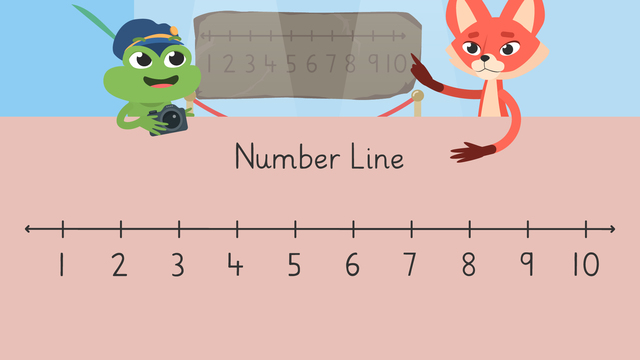# Using and Drawing Number LinesRating

Ø 5.0 / 1 ratings

The authorsTeam Digital

## Basics on the topicUsing and Drawing Number Lines

### In This Video About the Number Line Diagram

Skylar and Henry are visiting the famous math museum, The Hall of Numbers. As they are standing near the world’s oldest number line, Henry sneezes which causes him to jump and accidentally knock it down!! Now they need to make a new one before anybody notices! In this video,Skylar and Henry learn all about using and drawing number lines.

### What is a Number Line Diagram?

#### Number Line Diagram: A Definition

There are two types of number lines: closed number lines and open number lines. Both types are usually shown horizontally.Closed number lines are straight lines that show a set of numbers at equally spaced points called intervals. Arrows go on either end of the line to represent all the other numbers that we cannot see. Closed number lines can help us count, order, or find missing numbers. This is what is a closed number line used for.Open number lines are also straight lines that show numbers, but they start off blank and we fill in the numbers at the needed spaces, or intervals.The intervals can be made by small, medium or large jumps of one, five, ten, or one hundred. This is how an open number line works. ​​Open number lines help us when we are trying to solve problems by counting forward or backward with large numbers.On both numbers lines, numbers get larger when we move to the right or forward. Clue words like addition, plus, more, count on, or sum give us hints to move to the right. On both numbers lines, numbers get smaller when you move to the left or backward. Clue words like subtraction, minus, less, count back, or difference give us hints to move to the left.#### Drawing a Closed Number Line Diagram

First, draw a straight line with arrows on either end.Next, make marks at equal spaces along the line. To show the numbers one through ten, make ten marks.Then, find the starting number; here it is one. Label that on the left side.Finally, label all of the marks by counting up to the final marker; here it is ten.### Summary of Steps: Making a Closed Number Line Diagram

To draw a closed number line:

• First, draw a straight line with arrows on either end.

• Next, make marks at equal spaces along the line.

• Then, find the starting number and label that on the left side.

• Finally, label all of the marks by counting up to the final marker.

### Practice: Number Line Diagram 2nd Grade

Have you practiced yet? On this website, you can also find number line diagram worksheets and exercises.

### TranscriptUsing and Drawing Number Lines

Henry and Skylar are visiting a friend at Yosemite Tower. But, there is a problem! A buffalo moved in and every time he walks down the hall, the apartment numbers fall off the doors! The numbers are all out of order and they can't find their friend! Let's learn about "Ordering Numbers Up toTwenty" to help! When we talk about order, we mean listing things in a certain way. The numbers one through twenty follow a certain list when counting. It goes like this. If you see the numbers in different places, it's called out of order. To help us order numbers up to twenty, we can use a number line as a guide. Let's show Skylar how to use a number line to order apartments one through ten. Five apartment numbers are on the floor. But these apartments still have their numbers on their doors. Let's start by comparing the line of doors to a number line. Start by matching them up. Then, find the first blank door and look at the number line below. The number line says two, so we need to find the number two and put it here. Now, move to the next blank door and check the number line below. The number line below says three, that means the number three will go here. Let's look at the next blank doors and check them with the number line below. The number line shows us that five belongs here, eight belongs here and ten belongs here. Let's put the numbers back in their places! Now that the numbers are all back in order, let's count them to check our work! One, two, three, four, five, six, seven, eight, nine, ten! Great job, Skylar! Now let's help Henry. These are apartments eleven through twenty. Four apartments have numbers on the floor and five still have numbers on the doors. Let's check with a number line to find where each number belongs. Start by matching them up. Then find the blank doors and look for those places on the number line below. What numbers will we need? We will need eleven, thirteen, sixteen and nineteen! Now we can put the numbers back where they belong and count to make sure the order is correct! Eleven, twelve, thirteen, fourteen, fifteen, sixteen, seventeen, eighteen, nineteen, twenty! While Henry and Skylar find their friend's apartment, let's review! Today we learned about ordering numbers through twenty by using a number line. First, put a number line below the list with missing numbers. Next, look at the missing spaces and compare them to the number line below. Then, write the correct numbers in their spots. Finally, count the order to check your work! "I'm glad we got everything sorted but this can't keep happening!" "You're right, Henry. I have an idea." "No more stomping down the hall now!"